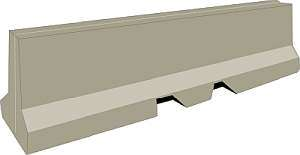# Feet and Inches to Centimeter Calculator

Instructions: Use this calculator to convert a lengths or heights given in feet+inches notation, showing all the steps. Please type a length you would like to convert in the form below such 6'2", etc:Type a length in feet and inches (Ex: 6' 1")

## Using this Feet+Inches to Centimeters Conversion Calculator

One common way of expressing lengths and heights in Anglo countries is by using what is called the imperial unit systems, that uses feet and inches as a basis for measuring extensions.

For example, a height given in imperial notation is something like 6' 2", which means 6 feet and 2 inch. And naturally, people in countries where the metric system is dominant (that uses meters and centimeters) would wonder, how much is 6' 2" in centimeters.## How convert feet+inch measurements into the metric system?

When you have something like 6' 2", you have an implicit addition of two units. Indeed, you have 6 feet PLUS 2 inches, and that is the way to proceed to conduct any conversion.

Notice that another related commonly used conversion is to convert heights in feet and inches to inches, and possibly convert imperial units to centimeters.

## How do you height conversion for imperial units

The idea is to separate the feet and inches parts, and use a conversion formula for them separately.

### Steps for the conversion of feet+inches to centimeters

1. Notice that 1 inch is the same as 2.54 centimeters and 1 foot is the same as 30.48 centimeters. This is used as your initial proportion
2. Assume you have a length or height given in feet+inches format. Identify the number of feet F, and the number of inches I
3. Using direct proportion, we establish that $$\displaystyle \frac{1}{2.54} = \frac{I}{C_1}$$ and $$\displaystyle \frac{1}{30.48} = \frac{F}{C_2}$$
4. Solving the proportion for $$C_1$$ and $$C_2$$, we get the following conversion formula for centimeters: $$C = C_1 + C_2 = 30.48 F + 2.54 I$$

Then, with this formula, you need to plug in the number of feet and inches to compute the equivalent number of centimeters.### Examples: Converting feet+inches to centimeters

What is 5'4 in cm?

Solution: We use the conversion formula:

$C = 30.48 F + 2.54 I$

In this case, the given length consists of 5 feet and 4 inches, so then plugging it in the above formula we find that :

$C = 30.48 F + 2.54 I = 30.48 \times 5 + 2.54 \times 4 = 162.56 \text{ cms}$

### Another conversion example

Convert 5'2 in cm

Solution: We can use the same exact procedure. We need to use the conversion formula:

$C = 30.48 F + 2.54 I$

and now the given length is 5' 2, which corresponds to 5 feet and 2 inches, so we find that:

$C = 30.48 F + 2.54 I = 30.48 \times 5 + 2.54 \times 2 = 157.48 \text{ cms}$

### More units conversions

Convert 5'11 in cm

Solution: We get the well known conversion formula from imperial units to centimeters:

$C = 30.48 F + 2.54 I$

In this case, 5' 11" is decomposed as 5 feet and 11 inches. Therefore, so we find that:

$C = 30.48 F + 2.54 I = 30.48 \times 5 + 2.54 \times 11 = 180.34 \text{ cms}$

## Conversion calculators

Consider the following calculators that also involve metric unit conversions, such as our meters to inches calculator, or the centimeters to feet calculator, or also our meters to feet, or imperial to meters calculators, that can help you with different conversion calculations.